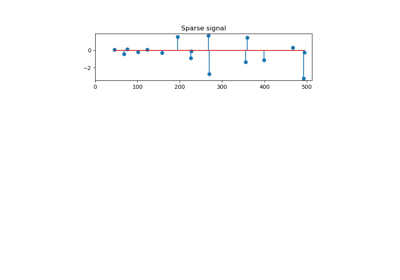/scikit-learn

# sklearn.linear_model.OrthogonalMatchingPursuit

`class sklearn.linear_model.OrthogonalMatchingPursuit(n_nonzero_coefs=None, tol=None, fit_intercept=True, normalize=True, precompute=’auto’)` [source]

Orthogonal Matching Pursuit model (OMP)

Read more in the User Guide.

Parameters: `n_nonzero_coefs : int, optional` Desired number of non-zero entries in the solution. If None (by default) this value is set to 10% of n_features. `tol : float, optional` Maximum norm of the residual. If not None, overrides n_nonzero_coefs. `fit_intercept : boolean, optional` whether to calculate the intercept for this model. If set to false, no intercept will be used in calculations (e.g. data is expected to be already centered). `normalize : boolean, optional, default True` This parameter is ignored when `fit_intercept` is set to False. If True, the regressors X will be normalized before regression by subtracting the mean and dividing by the l2-norm. If you wish to standardize, please use `sklearn.preprocessing.StandardScaler` before calling `fit` on an estimator with `normalize=False`. `precompute : {True, False, ‘auto’}, default ‘auto’` Whether to use a precomputed Gram and Xy matrix to speed up calculations. Improves performance when `n_targets` or `n_samples` is very large. Note that if you already have such matrices, you can pass them directly to the fit method. `coef_ : array, shape (n_features,) or (n_targets, n_features)` parameter vector (w in the formula) `intercept_ : float or array, shape (n_targets,)` independent term in decision function. `n_iter_ : int or array-like` Number of active features across every target.

#### Notes

Orthogonal matching pursuit was introduced in G. Mallat, Z. Zhang, Matching pursuits with time-frequency dictionaries, IEEE Transactions on Signal Processing, Vol. 41, No. 12. (December 1993), pp. 3397-3415. (http://blanche.polytechnique.fr/~mallat/papiers/MallatPursuit93.pdf)

This implementation is based on Rubinstein, R., Zibulevsky, M. and Elad, M., Efficient Implementation of the K-SVD Algorithm using Batch Orthogonal Matching Pursuit Technical Report - CS Technion, April 2008. http://www.cs.technion.ac.il/~ronrubin/Publications/KSVD-OMP-v2.pdf

#### Examples

```>>> from sklearn.linear_model import OrthogonalMatchingPursuit
>>> from sklearn.datasets import make_regression
>>> X, y = make_regression(noise=4, random_state=0)
>>> reg = OrthogonalMatchingPursuit().fit(X, y)
>>> reg.score(X, y)
0.9991...
>>> reg.predict(X[:1,])
array([-78.3854...])
```

#### Methods

 `fit`(X, y) Fit the model using X, y as training data. `get_params`([deep]) Get parameters for this estimator. `predict`(X) Predict using the linear model `score`(X, y[, sample_weight]) Returns the coefficient of determination R^2 of the prediction. `set_params`(**params) Set the parameters of this estimator.
`__init__(n_nonzero_coefs=None, tol=None, fit_intercept=True, normalize=True, precompute=’auto’)` [source]
`fit(X, y)` [source]

Fit the model using X, y as training data.

Parameters: `X : array-like, shape (n_samples, n_features)` Training data. `y : array-like, shape (n_samples,) or (n_samples, n_targets)` Target values. Will be cast to X’s dtype if necessary `self : object` returns an instance of self.
`get_params(deep=True)` [source]

Get parameters for this estimator.

Parameters: `deep : boolean, optional` If True, will return the parameters for this estimator and contained subobjects that are estimators. `params : mapping of string to any` Parameter names mapped to their values.
`predict(X)` [source]

Predict using the linear model

Parameters: `X : array_like or sparse matrix, shape (n_samples, n_features)` Samples. `C : array, shape (n_samples,)` Returns predicted values.
`score(X, y, sample_weight=None)` [source]

Returns the coefficient of determination R^2 of the prediction.

The coefficient R^2 is defined as (1 - u/v), where u is the residual sum of squares ((y_true - y_pred) ** 2).sum() and v is the total sum of squares ((y_true - y_true.mean()) ** 2).sum(). The best possible score is 1.0 and it can be negative (because the model can be arbitrarily worse). A constant model that always predicts the expected value of y, disregarding the input features, would get a R^2 score of 0.0.

Parameters: `X : array-like, shape = (n_samples, n_features)` Test samples. For some estimators this may be a precomputed kernel matrix instead, shape = (n_samples, n_samples_fitted], where n_samples_fitted is the number of samples used in the fitting for the estimator. `y : array-like, shape = (n_samples) or (n_samples, n_outputs)` True values for X. `sample_weight : array-like, shape = [n_samples], optional` Sample weights. `score : float` R^2 of self.predict(X) wrt. y.
`set_params(**params)` [source]

Set the parameters of this estimator.

The method works on simple estimators as well as on nested objects (such as pipelines). The latter have parameters of the form `<component>__<parameter>` so that it’s possible to update each component of a nested object.

Returns: self

## Examples using `sklearn.linear_model.OrthogonalMatchingPursuit`Orthogonal Matching Pursuit